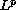Electron. J. Diff. Eqns., Vol. 2003(2003), No. 46, pp. 1-31.

### On uniqueness and existence of entropy solutions of weakly coupled systems of nonlinear degenerate parabolic equations Helge Holden, Kenneth H. Karlsen, & Nils H. Risebro

Abstract:
We prove existence and uniqueness of entropy solutions for the Cauchy problem of weakly coupled systems of nonlinear degenerate parabolic equations. We prove existence of an entropy solution by demonstrating that the Engquist-Osher finite difference scheme is convergent and that any limit function satisfies the entropy condition. The convergence proof is based on deriving a series of a priori estimates and using a generalcompactness criterion. The uniqueness proof is an adaption of Kruzkov's ``doubling of variables'' proof. We also present a numerical example motivated by biodegradation in porous media.

Submitted October 18, 2002. Published April 22, 2003.
Math Subject Classifications: 35K65, 65M12, 35L65.
Key Words: Nonlinear degenerate parabolic equations, weakly coupled systems, entropy solution, uniqueness, existence, finite difference method

Show me the PDF file (749K), TEX file, and other files for this article.Helge Holden Department of Mathematical Sciences Norwegian University of Science and Technology NO--7491 Trondheim, Norway email: holden@math.ntnu.no http://www.math.ntnu.no/~holden Kenneth H. Karlsen Department of Mathematics University of Bergen Johs. Brunsgt. 12 N-5008 Bergen, Norway e-mail: kennethk@mi.uib.no http://www.mi.uib.no/~kennethk Nils H. Risebro Department of Mathematics University of Oslo P.O. Box 1053, Blindern N-0316 Oslo, Norway e-mail: nilshr@math.uio.no http://www.math.uio.no/~nilshr